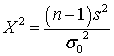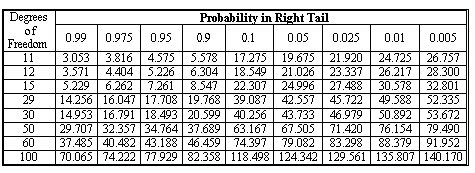### Why should I choose AnalystNotes?

Simply put: AnalystNotes offers the best value and the best product available to help you pass your exams.

##### Subject 11. Testing Concerning Tests of Variances (Chi-Square Test)

Suppose an analyst is interested in testing whether the variance from a single population is statistically equal to some hypothesized value. Let σ2 represent the variance and let σ02 represent the hypothesized value. The null and alternative hypotheses would be expressed as:

H0: σ2 = σ02, versus H1: σ2 ≠ σ02

H0: σ2 ≤ (σ0)2, versus H1: σ2 > (σ0)2, or H0: σ2 ≥ (σ0)2, versus H1: σ2 < (σ0)2

The test statistic to be used is a chi-square (χ2) statistic with n-1 degrees of freedom.

The formula for the test statistic is:n = sample size
s2 = sample variance
σ02 = the hypothesized value for σ under H0

Unlike t-graphs and z-graphs, a chi-square graph is positively skewed. It is also truncated at zero, and thus is not defined for negative values.

Like the family of t-graphs, the shape of the graph varies; the graph becomes more symmetrical as the degrees of freedom increase.

The graph looks like this:Critical values for this distribution are found in the chi-square table. The table is titled "Probability in Right Tail," so be aware that this is the region the table gives and modify your calculation accordingly.It is possible to use both tails in this distribution, despite the fact that it is non-symmetrical.

In contrast to the t-test, the chi-square test is sensitive to violations of its assumptions. If the sample is not actually random or if it does not come from a normally distributed population, inferences based on a chi-square test are likely to be faulty.

Example

You are an institutional investor evaluating a hedge fund that seeks to deliver a return comparable to the domestic broad market equity index while keeping the monthly standard deviation in asset value under 5%. According to data in the prospectus, during the last 30 months the monthly standard deviation in asset value was 4.5%. You wish to test this claim statistically, using a significance level of 0.10. Assume that returns are normally distributed and monthly asset values are independent observations.

The hypotheses are:

H0: σ2 ≤ 0.0025, versus H1: σ2 > 0.0025. Note that by squaring the standard deviation, 5% = 0.05, you get 0.0025.

The test statistic will be chi-square with 30 - 1 = 29 degrees of freedom.

The critical value will be found in the table for a chi-square distribution, 29 degrees of freedom, alpha = 0.10. Note that this is a one-tailed test. The critical value is 39.087.

The test statistic will be: chi-square = [(29) x 0.0452] / (0.05)2 = 23.49.

The test statistic is not greater than the critical value, so you do not reject the null hypothesis.

Note: If the null hypothesis had a >= symbol in it, then you would reject if the test statistic was less than or equal to the lower alpha point.

The chi-square distribution is asymmetrical. Like the t-distribution, the chi-square distribution is a family of distributions; a different distribution exists for each possible value of degrees of freedom, n - 1 (n is sample size). Unlike the t-distribution, the chi-square distribution is bounded below by 0 (no negative values).

User Comment
vincenthuang Chi-square=(n-1)*S2/sigma2
fiderikumo Why is Chi square the most appropriate test here?
tanyak because it is the test of variance
achu If we had made the H1 hypothesis [variance < .025 ] instead of [var> than .025] : Then H0 would have been (var) >= .025; Chi-squared value from table is 19.768 (since left tail = 100%- 90% in right tail), and H1 is var < .025. Because test stat is 23.49, Wouldn't that also be a 'do not reject' at 10% signficance level?
chamad Back to the example. I Understood from thr previous readings that Alternative Hyp Ha is what we try to proof. Shouldn't be Ha:var<0.025 and H0 : var>=0.025 ??? someone please?
SuperKnight I don't understand I would have thought that the null hypothesis would be opposite of what they show here? You are trying to prove the alternate hypothesis so I would figure you'd have to set the null hypothesis at var >= 0.0025, and then if you find that the 4.5% std dev is significantly lower than 5%, you could reject the null hypothesis and conclude something? Perhaps this difference has something to do with the fact that Ch-square graph is skewed to the right?
Capital No SuperKnight. You can reject null hypothesis. That's the purpose of hypothesis testing. However, you cannot accept therefore the alternative hypothesis.
SaeedAlam SuperKnight I'm sure you can do it your way, with reference to the third pargraph from the end. Just switch everything
gill15 I got all this stuff but I`m confused about setting the null and alternative hypothesis as well...dont get it....it`s the opposite of how we`ve been doing it
gill15 It doesnt seem to matter actually....your rejection or acceptance would just be opposite then ---- what i`m trying to say it looks like it doesnt matter what you choose your null to be...
Shaan23 I did the opposite way. Ho: o^2 > .0025 but I'm missing something.

Get the same test statistic = 23.49. My critical value is at the 90th percentile because I'm doing the Ha the other end of the chi graph which at 29 df is 19.768.

So I do not reject the null hypothesis but I should I right? to be compatible with the example.